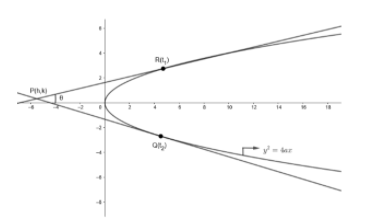QuestionAnswers

# Let $P$ be a point on the parabola ${{y}^{2}}-2y-4x+5=0$, such that the tangent on the parabola at $P$ intersects the directrix at a point $Q$. Let $R$ be the point that divides the line segment $QP$ externally in the ratio $\dfrac{1}{2}:1$. Find the locus of $R$.Hint: We use the formula for angle b/w two intersecting lines at a point, which is $\tan \theta =\dfrac{\left( {{m}_{1}}-{{m}_{2}} \right)}{1+{{m}_{1}}{{m}_{2}}}$ and find ${{m}_{1}}$and ${{m}_{2}}$ by equation of tangent.

We have been given a parabola ${{y}^{2}}=4ax$ such that tangents meet at an angle of $\theta$.
We have to prove that locus of points of intersection of given tangents is ${{\tan }^{2}}\theta {{\left( x+a \right)}^{2}}={{y}^{2}}-4ax$
Let the point of intersection be $P\left( h,k \right)$, which is as shown.Let us take two tangents at point $R\left( {{t}_{1}} \right)$ and point $Q\left( {{t}_{2}} \right)$ and they intersect at point $P\left( h,k \right)$.
We know that any general point on parabola ${{y}^{2}}=4ax$ is $\left( x,y \right)=\left( a{{t}^{2}},2at \right)$.
Therefore, we get point $R\left( {{t}_{1}} \right)=\left( at_{1}^{2},2a{{t}_{1}} \right)$ and point $Q\left( {{t}_{2}} \right)=\left( at_{2}^{2},2a{{t}_{2}} \right)$
Now, we know that any tangent at point $\left( a{{t}^{2}},2at \right)$ in parabola is $ty=x+a{{t}^{2}}$.
Therefore, tangent at point $R\left( {{t}_{1}} \right)\Rightarrow {{t}_{1}}y=x+at_{1}^{2}....\left( i \right)$
And tangent at point $Q\left( {{t}_{2}} \right)\Rightarrow {{t}_{2}}y=x+at_{2}^{2}....\left( ii \right)$
We are given that tangents at $R\left( {{t}_{1}} \right)$and $Q\left( {{t}_{2}} \right)$intersect at point $P\left( h,k \right)$.
So, now we will put $\left( h,k \right)$in equation $\left( i \right)$ and $\left( ii \right)$.
We get, ${{t}_{1}}k=h+at_{1}^{2}$
Or, $h={{t}_{1}}k-at_{1}^{2}....\left( iii \right)$
And ${{t}_{2}}k=h+at_{2}^{2}$
Or, $h={{t}_{2}}k-at_{2}^{2}....\left( iv \right)$
Now we equate $h$from equation $\left( iii \right)$and $\left( iv \right)$.
We get, ${{t}_{1}}k-at_{1}^{2}={{t}_{2}}k-at_{2}^{2}$
By rearranging the equation,
We get, $k\left( {{t}_{1}}-{{t}_{2}} \right)=a\left( t_{1}^{2}-t_{2}^{2} \right)$
Since we know that ${{a}^{2}}-{{b}^{2}}=\left( a-b \right)\left( a+b \right)$
Therefore, we get $k\left( {{t}_{1}}-{{t}_{2}} \right)=a\left( {{t}_{1}}-{{t}_{2}} \right)\left( {{t}_{1}}+{{t}_{2}} \right)$
By cancelling like terms we get,
$\dfrac{k}{a}=\left( {{t}_{1}}+{{t}_{2}} \right)....\left( v \right)$
Now we will put the value of $k$ in equation $\left( iii \right)$ to get the value of $h$, that is:
$h={{t}_{1}}\left[ a\left( {{t}_{1}}+{{t}_{2}} \right) \right]-at_{1}^{2}$
$h=at_{1}^{2}+a{{t}_{1}}{{t}_{2}}-at_{1}^{2}$
Therefore, we get $\dfrac{+h}{a}={{t}_{1}}{{t}_{2}}....\left( vi \right)$
Now, we know that two lines having slopes ${{m}_{1}}$and ${{m}_{2}}$ and containing angle $\theta$ between them would satisfy $\tan \theta =\left| \dfrac{\left( {{m}_{1}}-{{m}_{2}} \right)}{1+\left( {{m}_{1}}{{m}_{2}} \right)} \right|....\left( vii \right)$
Since, tangent at $R\left( {{t}_{1}} \right)$ which is $y=\dfrac{1}{{{t}_{1}}}x+a{{t}_{1}}$ and tangent at $Q\left( {{t}_{2}} \right)$which is $y=\dfrac{1}{{{t}_{2}}}x+a{{t}_{2}}$ have angle $\theta$between them. Therefore, their slopes will satisfy the equation $\left( vii \right)$.
Comparing with $y=mx+c$
Tangent at $R\left( {{t}_{1}} \right)$ would have slope ${{m}_{1}}=\dfrac{1}{{{t}_{1}}}$and tangent at $Q\left( {{t}_{2}} \right)$ would have slope ${{m}_{2}}=\dfrac{1}{{{t}_{2}}}$.
Now, we will put these values in equation $\left( vii \right)$.
We get, $\tan \theta =\left| \dfrac{\dfrac{1}{{{t}_{1}}}-\dfrac{1}{{{t}_{2}}}}{1+\dfrac{1}{{{t}_{1}}}.\dfrac{1}{{{t}_{2}}}} \right|$
As the above result contains the modulus function, we will square both the sides to get a neutral equation.
We get, ${{\tan }^{2}}\theta ={{\left( \dfrac{\dfrac{1}{{{t}_{1}}}-\dfrac{1}{{{t}_{2}}}}{1+\dfrac{1}{{{t}_{1}}}.\dfrac{1}{{{t}_{2}}}} \right)}^{2}}$
By simplifying the equation,
We get, $\Rightarrow {{\tan }^{2}}\theta =\dfrac{{{\left( -{{t}_{1}}+{{t}_{2}} \right)}^{2}}}{{{\left( {{t}_{1}}{{t}_{2}}+1 \right)}^{2}}}$
Since, we know that ${{\left( {{t}_{2}}-{{t}_{1}} \right)}^{2}}={{\left( {{t}_{1}}-{{t}_{2}} \right)}^{2}}$
Therefore, ${{\tan }^{2}}\theta =\dfrac{{{\left( {{t}_{1}}-{{t}_{2}} \right)}^{2}}}{{{\left( 1+{{t}_{1}}{{t}_{2}} \right)}^{2}}}$
Since, we know that ${{\left( a-b \right)}^{2}}={{a}^{2}}+{{b}^{2}}-2ab$and ${{\left( a+b \right)}^{2}}={{a}^{2}}+{{b}^{2}}+2ab$
We get ${{\tan }^{2}}\theta =\dfrac{t_{1}^{2}+t_{2}^{2}-2{{t}_{1}}{{t}_{2}}}{1+{{\left( {{t}_{1}}{{t}_{2}} \right)}^{2}}+2{{t}_{1}}{{t}_{2}}}$
By adding and subtracting $2{{t}_{1}}{{t}_{2}}$in numerator,
We get, ${{\tan }^{2}}\theta =\dfrac{t_{1}^{2}+t_{2}^{2}+2{{t}_{1}}{{t}_{2}}-2{{t}_{1}}{{t}_{2}}-2{{t}_{1}}{{t}_{2}}}{1+{{\left( {{t}_{1}}{{t}_{2}} \right)}^{2}}+2{{t}_{1}}{{t}_{2}}}$
Or, ${{\tan }^{2}}\theta =\dfrac{{{\left( {{t}_{1}}+{{t}_{2}} \right)}^{2}}-4{{t}_{1}}{{t}_{2}}}{1+{{\left( {{t}_{1}}{{t}_{2}} \right)}^{2}}+2{{t}_{1}}{{t}_{2}}}$
Now, we will put the values of $\left( {{t}_{1}}+{{t}_{2}} \right)$ and ${{t}_{1}}{{t}_{2}}$ from equation $\left( v \right)$ and $\left( vi \right)$ respectively.
We get ${{\tan }^{2}}\theta =\dfrac{{{\left( \dfrac{k}{a} \right)}^{2}}-4\left( \dfrac{k}{a} \right)}{1+{{\left( \dfrac{h}{a} \right)}^{2}}+\dfrac{2h}{a}}$
By simplifying the equation and cancelling the like terms,
We get, ${{\tan }^{2}}\theta =\dfrac{{{k}^{2}}-4ha}{{{a}^{2}}+{{h}^{2}}+2ha}$
By cross multiplying the above equation, we get
${{\tan }^{2}}\theta \left( {{h}^{2}}+{{a}^{2}}+2ha \right)={{k}^{2}}-4ha$
Since we know that, $\left[ {{a}^{2}}+{{b}^{2}}+2ab={{\left( a+b \right)}^{2}} \right]$
We get, ${{\tan }^{2}}\theta {{\left( h+a \right)}^{2}}={{k}^{2}}-4ha$
Now, to get the locus, replace $h$by $x$ and $k$by $y$.
Hence, we get ${{\tan }^{2}}\theta {{\left( x+a \right)}^{2}}={{y}^{2}}-4ax$
Hence Proved.

Note: Students must take care of squaring the equation in case of modulus function. Also must draw the diagram first in such types of questions to understand the approach of solving. Take special care of signs of various variables to avoid mistakes.
View Notes
Equation of ParabolaParabola GraphPerpendicular Distance Of A Point From A PlaneMagnetic and Electric Force on a Point ChargeTable of 50 - Multiplication Table of 50Factors of 50Line Equation Point SlopeTangent of a CircleIntercept Form of the Equation of a PlaneCo-ordinate of a Point in Three Dimension